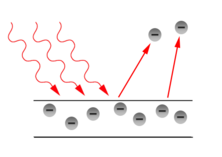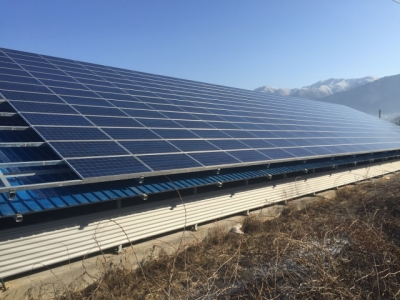# Photoelectric Effect 2

Photoelectric Effect

The photoelectric effect is a phenomenon where electrons pop out when a light beam is incident on a metal surface.
Simply think, the energy of light is transformed into the form of electrical energy.
However, electrons will only be emitted when the light is incident on the metal at a certain frequency or higher. The minimum photon energy at which electrons can stick out is the ‘work function’.
Usually, alkali metals such as sodium (Na) are often used in photoelectric effect experiments. This is because the work function of alkali metals is small, so it is easy to cause photoelectric effect even with low frequency visible light.Energy of one photon

Light (electromagnetic waves) is both a wave and a particle.
If light (photons) at the same frequency, the energy carried by the light (photons) is the same.
Light moves like individual particles, and half particles do not exist.The amount of energy carried by a single light particle (photon) is:

$E = h\nu$

h is Planck’s constant (6.626×10-34J·s), and ν(nu) is the frequency of light (s-1). If the speed of light is c(299,792,458 m/s),

$\nu =\frac { c }{ \lambda }$

You can also use the energy size of a photon as :

$E=\frac { hc }{ \lambda }$

λ(rambda) is the wavelength of light in meters

Kinetic energy of photoelectron and ‘stopping Voltage’

In a photoelectric effect experiment device such as the simulation above, when monochromatic light shines on a metal plane, electrons are released due to the photoelectric effect on the metal plate.

At this time, if a voltage is applied between the anode and the cathode to interfere with the movement of the emitted electrons, the kinetic energy of the emitted electrons decreases and eventually the electrons will not be released.
This voltage is called the ‘stopping voltage’.

In other words, the maximum kinetic energy Kmax of the electrons released without stopping voltage is equal to the stopping voltage multiplied by the amount of electron charge.

${ K }_{ max }\, =\, eV$

In addition, the metal surface itself may interfere with the emission of electrons. This energy is the ‘work function’.Therefore, if the stopping voltage is not applied, the kinetic energy of the emitted electrons can be obtained as follows.

${ K }_{ max }\, =\, h\nu \, -\, W$

When stopping voltage is applied, the maximum kinetic energy of emitted electrons

When the stopping voltage is applied, the maximum kinetic energy Kmax of the emitted electron is calculated as follows.

${ K }_{ max }\, =\, h\nu \, – \, W \, – \, (Energy loss due to stopping voltage)$

Convert unit J of energy to eV

In terms of the photoelectric effect, it is convenient to change the unit of energy to eV.1 eV is the amount of energy when one electron is accelerated at a potential of 1V.

$1eV \, = \, 1.6×{ 10 }^{ -19 }J$

Future energy source, the sun

This photoelectric effect becomes the basic concept of solar power generation. In other words, when light is emitted above a certain frequency, electrons pop out immediately (no delay in time).
If these electrons are allowed to run through electrical circuits, then solar power is generated.
Solar cells are semiconductors made from silicon (Si) in the sand. When sunlight shines on a solar cell, the electrons in it move and flow along the wires, and the flow of electrons creates electrical energy.Solar cells are made by adding very small amounts of phosphorus and boron to each side of thin silicon plates, which are commonly used as semiconductors.
Most commercially available silicon solar cells can convert 15 to 25 percent of the energy contained in sunlight into electricity.
The output voltage of one solar panel is only about 0.6V. So we connect several plates together to increase the output voltage and current.